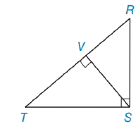Chapter 5.4, Problem 4E### Elementary Geometry for College St...

6th Edition
Daniel C. Alexander + 1 other
ISBN: 9781285195698

#### Solutions

Chapter
Section### Elementary Geometry for College St...

6th Edition
Daniel C. Alexander + 1 other
ISBN: 9781285195698
Textbook Problem
3 views

# Use theorem 5.4.3 to form a proportion in which TS is a geometric mean.[Hint: Δ T V S ∼ Δ T S R ]Exercises 1-6

To determine

To Find:

Proportion in which TS is the geometric mean.

Explanation

Given:

From the figure,

ΔRST is a right triangle with right angle RST, and SV¯ is the altitude to hypotenuse TR¯.

From the theorem,

The length of the altitude to the hypotenuse of a right triangle is the geometric mean of the lengths of the segments of the hypotenuse.

Since ΔRVSΔRST

Corresponding sides of similar triangles are proportional (CSSTP)

So, we have

VTTS=TSRT

Recall the Means-Extremes Property

In a proportion, the product of the means equals the product of the extremes

### Still sussing out bartleby?

Check out a sample textbook solution.

See a sample solution

#### The Solution to Your Study Problems

Bartleby provides explanations to thousands of textbook problems written by our experts, many with advanced degrees!

Get Started

#### Consider the data 10, 12, 12, 8, 30, 12, 8, 10, 5, 23. 2. Find the median.

Mathematical Applications for the Management, Life, and Social Sciences

#### Rewritten as an iterated integral in polar coordinates,

Study Guide for Stewart's Multivariable Calculus, 8th

#### Graph each function. fx=x-13-3

College Algebra (MindTap Course List)

#### Define a construct and explain the role that constructs play in theories.

Research Methods for the Behavioral Sciences (MindTap Course List)